Function Repository Resource:

# FociPointEllipse

Find the equation for an ellipse given two foci and a point

Contributed by: Ed Pegg Jr and Jan Mangaldan
 ResourceFunction["FociPointEllipse"][{f1,f2,p},{x,y}] returns the ellipse A x2+B x y+C y2+D x+E y+F in the variables x and y, given the foci f1,f2 and a point p through which the ellipse passes. ResourceFunction["FociPointEllipse"][{f1,f2,p},t] returns a parametric equation in the variable t. ResourceFunction["FociPointEllipse"][{f1,f2,p}] returns an Ellipsoid object representing the ellipse.

## Examples

### Basic Examples (2)

Find the Cartesian equation of an ellipse with foci (3,3) and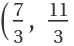) that goes through point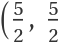):

 In:=Out=Show the ellipse:

 In:=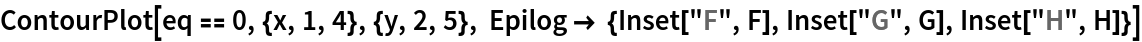Out=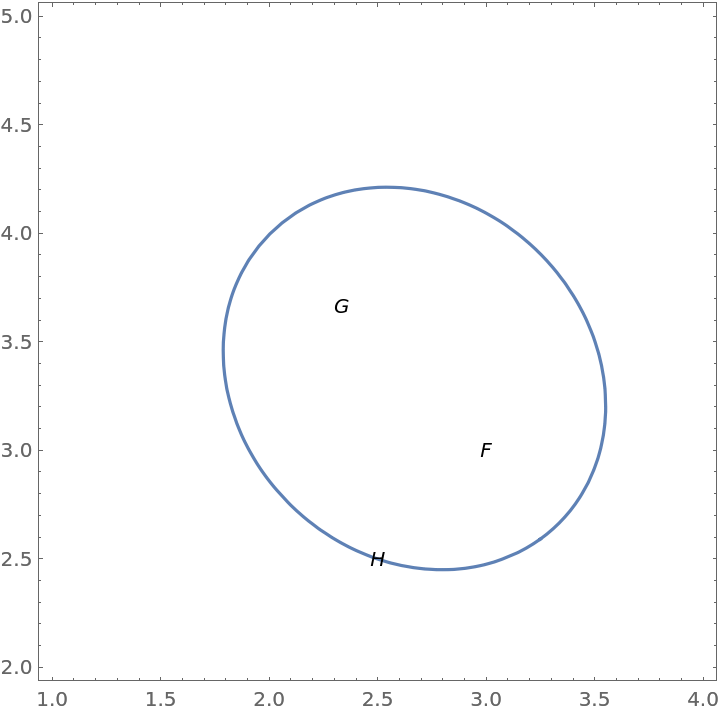### Scope (4)

Generate the parametric equations of an ellipse with foci (3,3) and) that goes through point):

 In:=Out=Show the ellipse:

 In:=Out=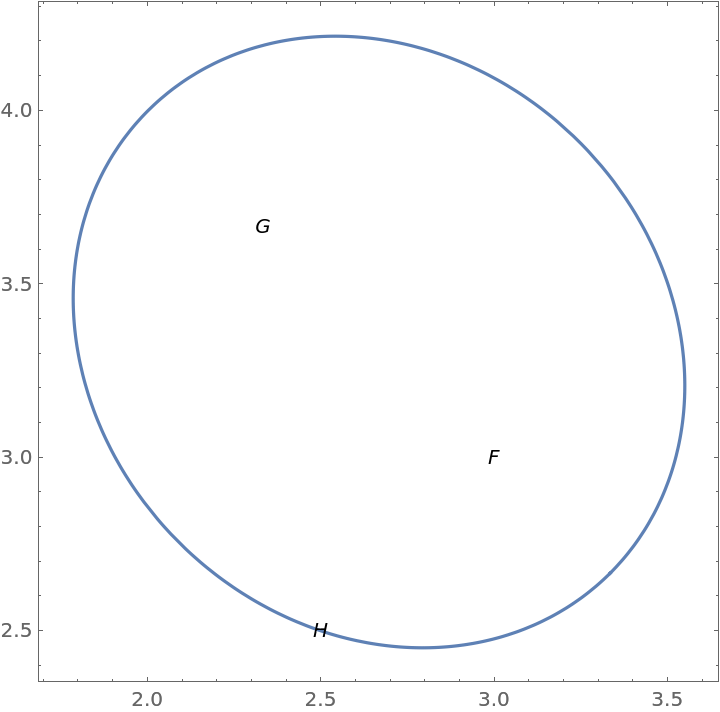Generate the corresponding Ellipsoid object:

 In:=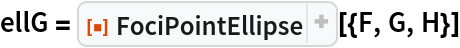Out=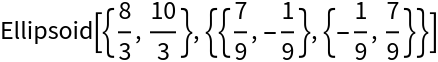Show the ellipse:

 In:=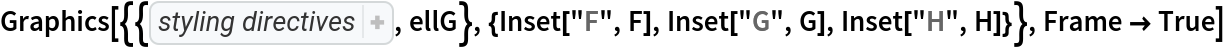Out=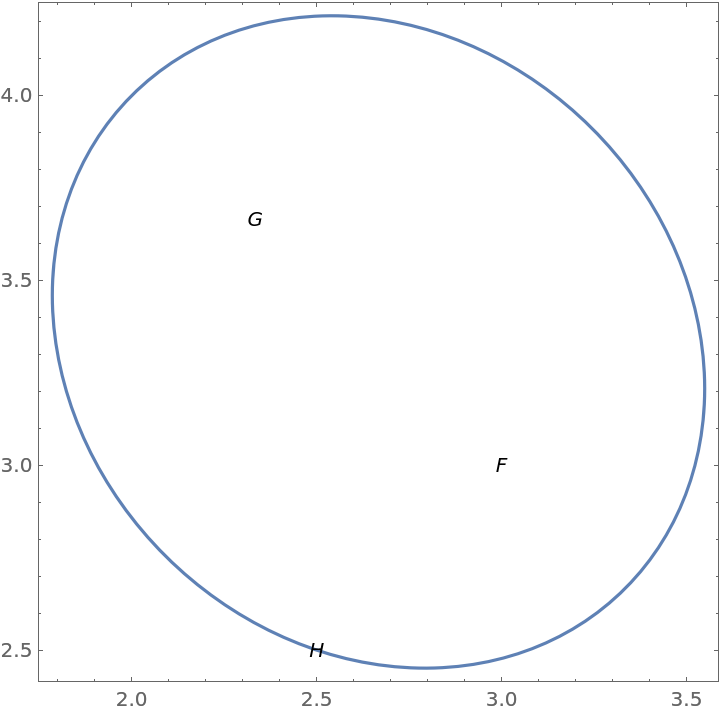### Properties and Relations (4)

Use a different set of variables:

 In:=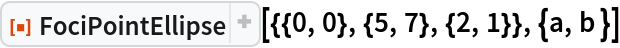Out=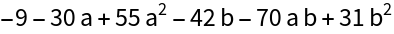Use formal variables:

 In:=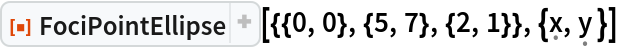Out=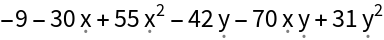Use FociPointEllipse to generate the implicit Cartesian equation of an ellipse:

 In:=Out=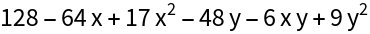Use GroebnerBasis to get an equivalent result:

 In:=Out=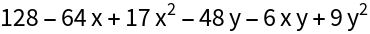Generate an equivalent parametric equation:

 In:=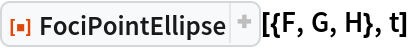Out=Use GroebnerBasis to derive the implicit Cartesian equation from the parametric equation:

 In:=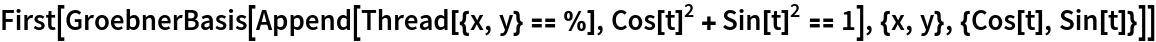Out=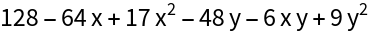Use the resource function EllipseProperties to generate properties of the ellipse:

 In:=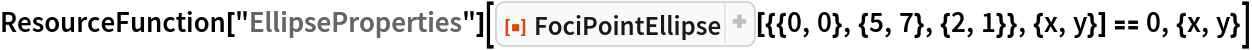Out=Get an ellipse equation:

 In:=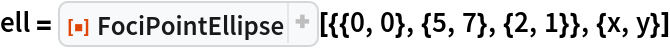Out=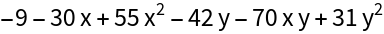Show positions for coefficients in A x2+B x y+C y2+D x+E y+F=0:

 In:=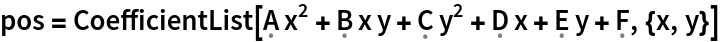Out=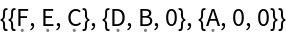Get the coefficients:

 In:=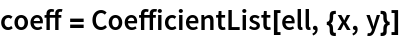Out=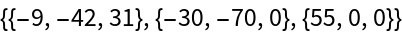See the coefficients in the standard order:

 In:=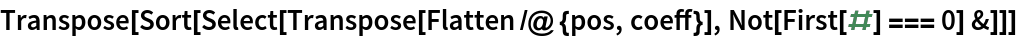Out=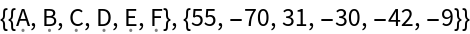### Neat Examples (4)

Construct an ellipse from given foci and a point:

 In:=Out=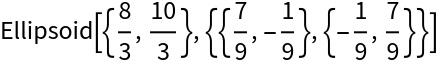Use three mysterious points to create a complex cubic, take the derivative and solve the quadratic to find foci that happen to be F and G:

 In:=Out=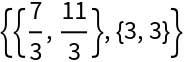In Marden's theorem, the above step finds the foci of an inellipse that is tangent to the midpoints of the sides of the triangle generated by vertices PQR:

 In:=Out=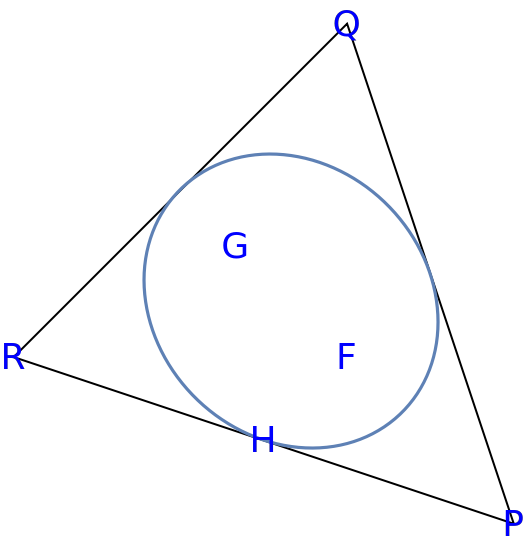The resulting inellipse is a scaled version of the Steiner circumellipse, with a scaling factor of 1/2. Use the resource function SteinerCircumellipse to produce an equivalent figure:

 In:=Out=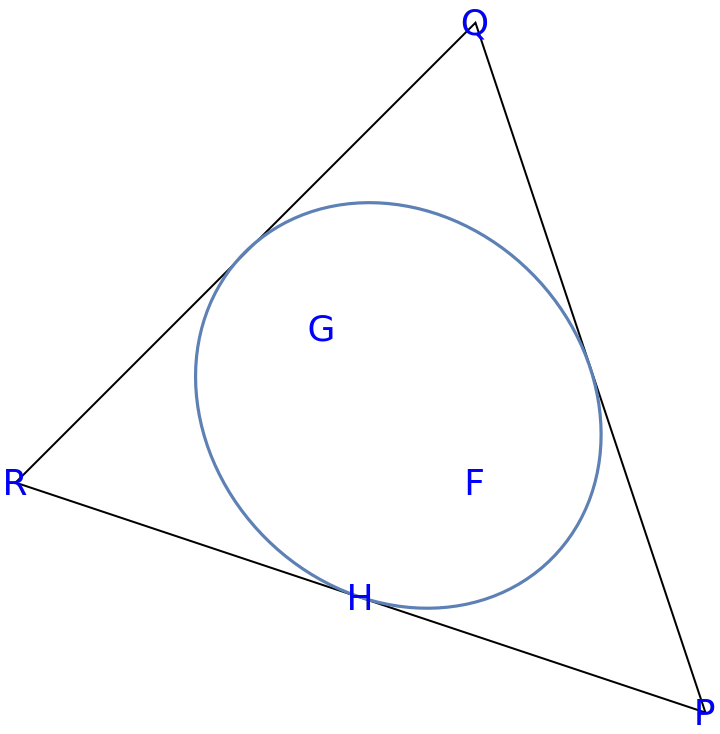## Version History

• 1.0.0 – 18 July 2022